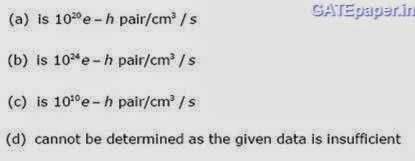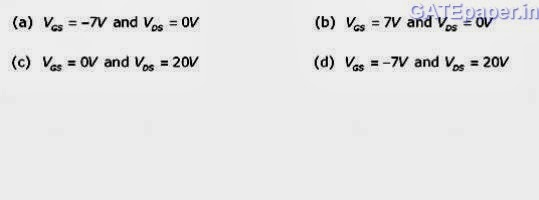## Search this site

### GATE 1992 EDC Video Solutions - Electronic Devices and Circuits

1.       A semiconductor is irradiated with light such that carriers are uniformly generated throughout its volume. The semiconductor is n-type with ND = 1019 cm-3. If the excess electron concentration in the steady state is Δn = 1015 cm-3 and if τp = 10 µsec (minority carrier life time), then the generation rate due to irradiation is ………….2.       A PN junction with a 100 resistor is forward biased so that a current of 100 mA flows. If the voltage across this combination is instantaneously reversed to 10 volts at t = 0, the reverse current that flows through the diode at t = 0 is approximately given by
a.       0 mA
b.      100 mA
c.       200 mA
d.      50 mA
3.       An infrared LED is usually fabricated from
a.       Ge
b.      Si
c.       GaAs
d.      GaAsP
4.       In a transistor having finite β, the forward bias across the base emitter junction is kept constant and the reverse bias across the collector base junction is increased. Neglecting the leakage across the collector base junction and the depletion region generating current, the base current will………….. (Increase / Decrease / Remains constant).
5.       An n-channel JFET has a pinch off voltage VP = -5 volts, VDSmax = 20 volts, and gm = 2 mA/V. The minimum ON resistance is achieved in the JFET for …………6.       The JFET in the circuit shown has an IDSS = 10 mA and VP = 5 volts. The value of the resistance RS for a drain current IDS of 6.4 mA is …………….. (Select the nearest value).7.       If the transistors in figure, have high values of β and a VBE of 0.65 volts, the current I flowing through the 2 K resistance will be…………8.       The 6 volts Zener diode shown in figure has zero zener resistance and a knee current of 5 mA. The minimum value of R so that the voltage across it does not fall below 6 volts is ……9.       A PNP transistor shown in figure has uniform doping in the emitter, base and collector regions, where in the doping concentrations are 1019 cm-3, 1017 cm-3 and 1015 cm-3 respectively. The minority carrier diffusion lengths in the emitter and the base regions are 5 µm and 100µm respectively.
Assuming low level injection conditions and using law of the junction, calculate the collector current density and the base current density due to base recombination.
[Suitable approximations may be made if required.]In all the regions of transistors, Dp = 8 cm2/sec, Dn = 16 cm2/sec, ni = 1.5 X 1010 cm-3, KT/q = 26 mV and q = 1.6  X 10-19 C.

Solution :

10.   An n-channel MOSFET having a threshold voltage of 2 volts is used in the circuit shown in figure. Initially the transistor is OFF and is in steady state. At time t = 0, a step voltage of magnitude of 4 volts is applied to the input so that the MOSFET turns ON instantaneously.
Draw the equivalent circuit and calculate the time taken to the output Vo to fall to 5 volts.
The device constant of the MOSFET, K = 5 mA/ V2, CDS =0 and CDG = 0.